Bucknell University // Assistant Professor of Computer Science## Introduction to Arduino Programming

April 16th, 2015 | Posted by in Uncategorized

The Arduino is an open-source electronics platform that is designed to be easy to program. In this project we will use the Arduino to build a rainbow thermometer. What is a rainbow thermometer? Simple; it senses the temperature and then lights up a colored LED (light emitting diode) to indicate the temperature. If you look at the rainbow colors they go from red to green to violet. When thinking about temperature, it is natural to use red to indicate hot, green to indicate just right, and blue (or violet) to indicate cold.## Goal

Our design goal is to sense the current temperature and then light up a LED. To keep things simple, we’ll assume the temperature we care about is from 0 C to 50 C (32 F to 112 F), with 0 C being dark blue, around 20 C green and 50 C dark red.

## Parts

 Arduino Nano (ATmega 328)10 K Ohm thermistor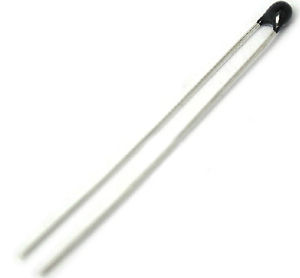RGB LED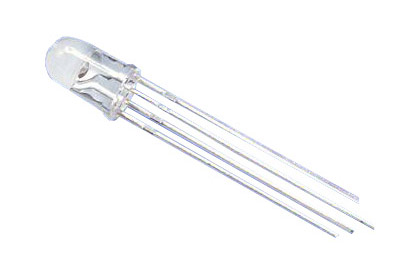100 Ohm resistor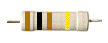10 KOhm resistor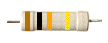Breadboard (and assorted wires)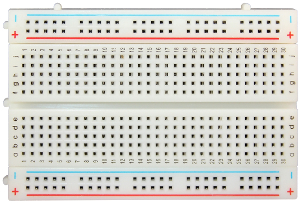If you have time, watch Massimo Banzi’s TED talk describing the Arduino project.

## Part 1: Blink

Before we get to work, it’s a good idea to make sure our Arduino and software is working correctly.

First, insert the Arduino into the breadboard. Be careful that all of the pins are in the breadboard and not the bend any pins.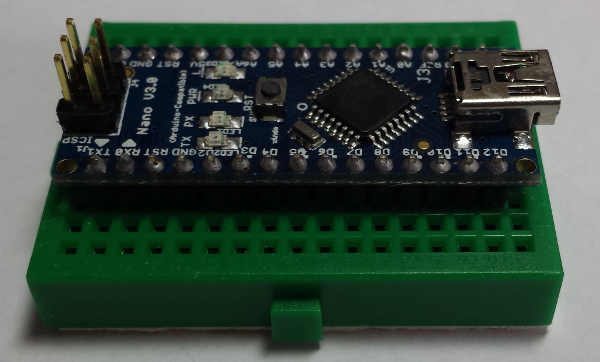Now connect the USB cable to your computer and open the Arduino software by typing “arduino” at a terminal. To open the terminal click theicon.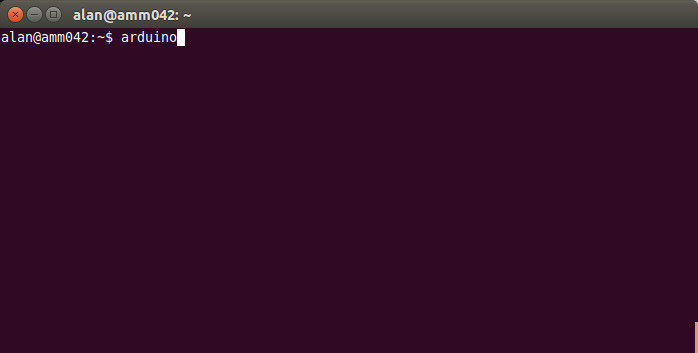This should open the Arduino interface similar to the one shown below.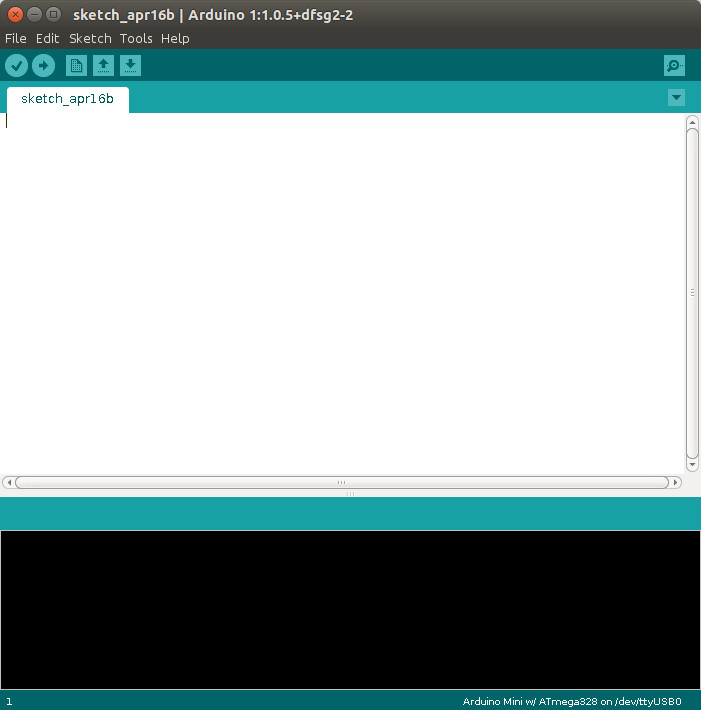### Now, select the board we are using (it is compatible with the Arduino Mini w/ ATmega328).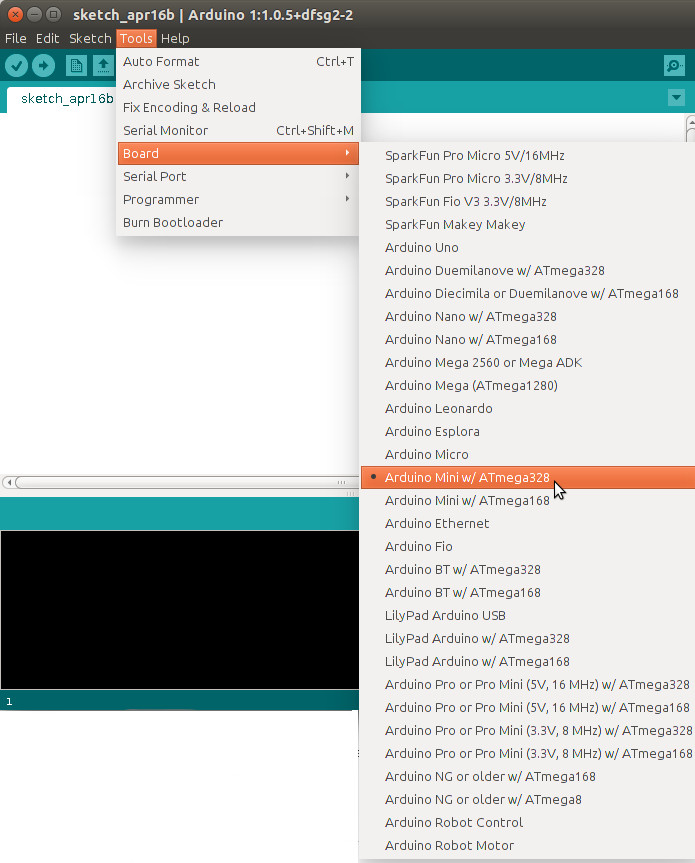And then check that the a port like /dev/ttyUSB0 is selected.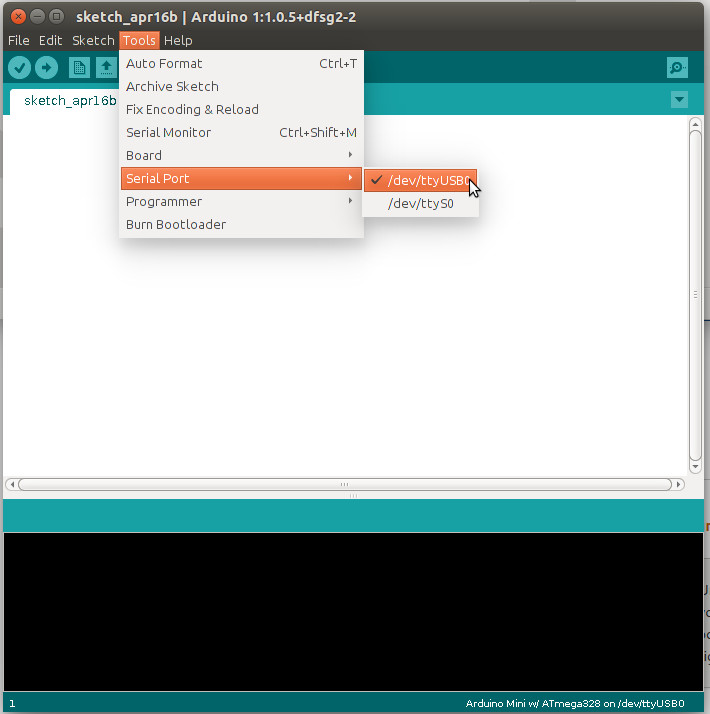On the bottom right of the Arduino window it should say “Arduino Mini w/ATmega328 on /dev/ttyUSB0”.

Now for a simple test program, select Examples->Basic->Blink.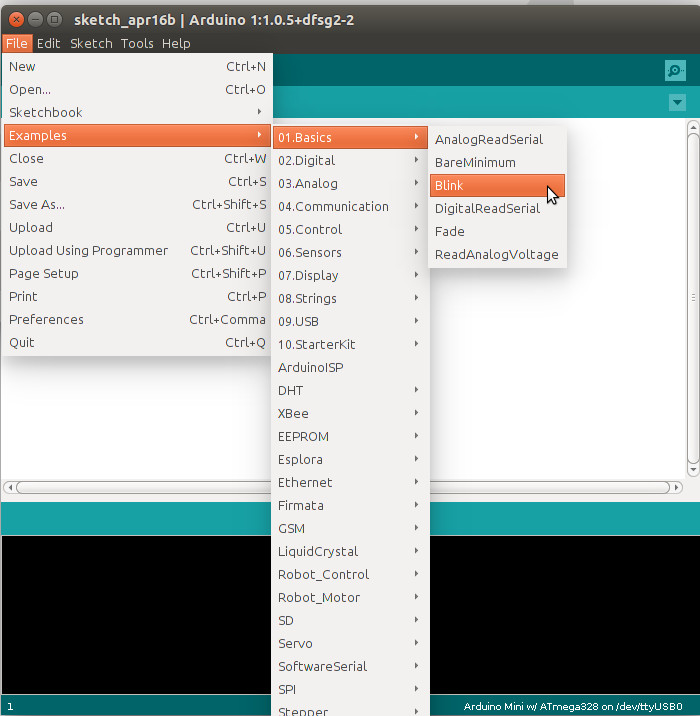This will open a new window with some code. You will notice to sections setup and loop. The setup code is run once when the Arduino turns on. In this case, it configures the LED pin for output. Then the loop code is run forever. It sets the output voltage high, waits 1 second (1000 milliseconds), sets the voltage low, and waits 1 second. The loop then repeats and the small red on-board LED should blink slowly. To run the program click the upload button.If your LED is not blinking slowly, get help now!

## Part 2: Temperature

To measure temperature, we are going to use a thermistor. A thermistor is a device that has a temperature-dependent change in resistance. To measure resistance we will construct a voltage divider using our 10 K Ohm resistor (brown-black-orange-gold bands). The circuit is shown below.To minimize self-heating we will only enable the sensor when we want to take a sample. To achieve this we will power the sensor from pin D13 and sample the voltage on pin A0. Connect the thermistor between these two pins. Then also connect a resistor from A0 to ground.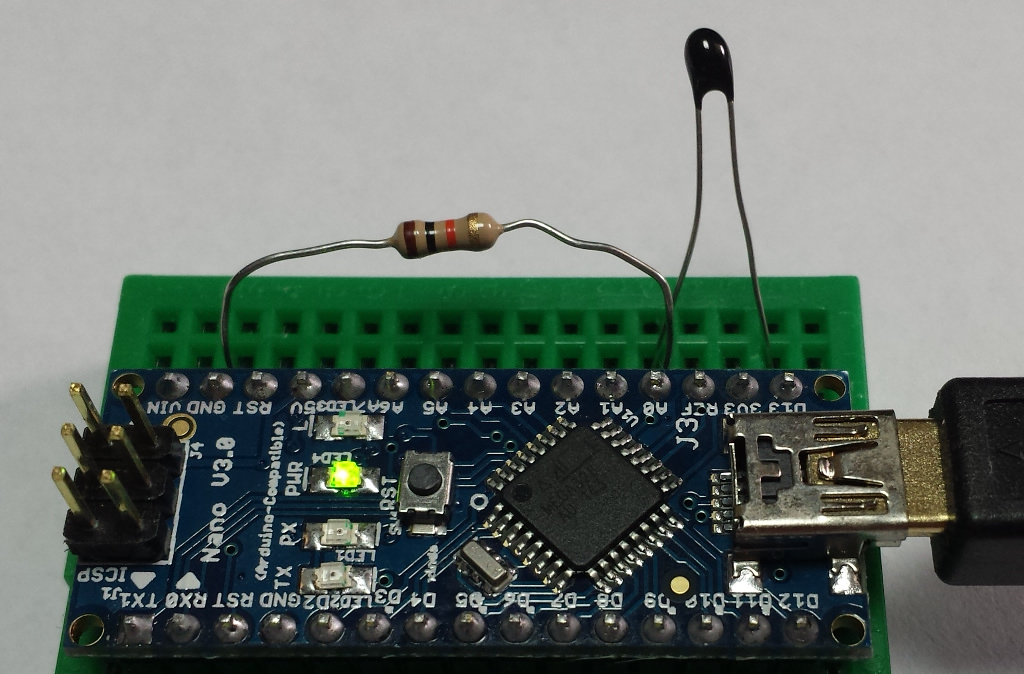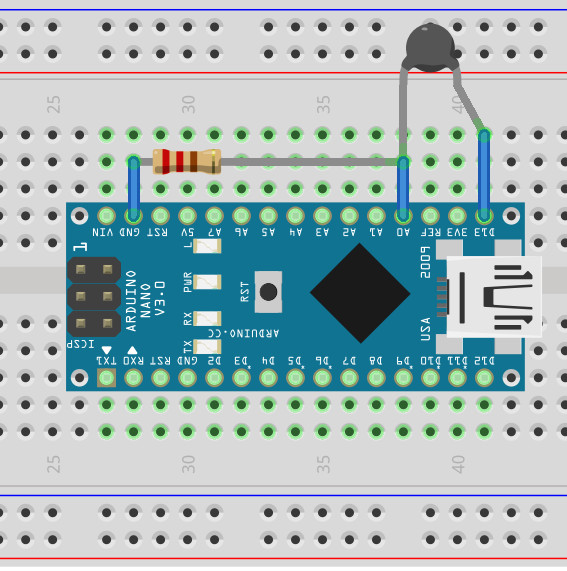The following program uses our helper library called RainbowThermometer. It is already installed on our computers, but you can also download it to look at the source. If you want to install it, unzip it into your libraries folder.

Upload this to your Arduino and then open the serial monitor as shown below.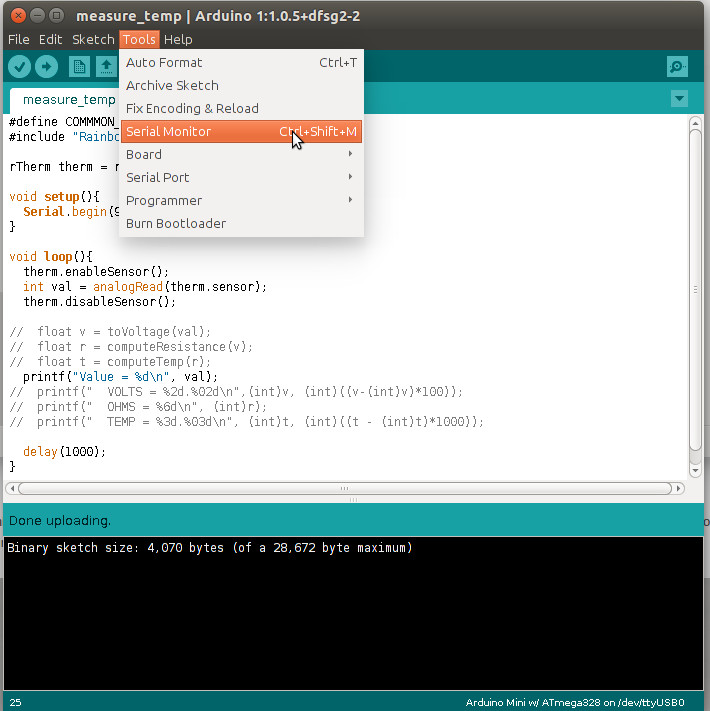This should open another window and after a little while (10 seconds or so), you will start seeing lines like:

This value is the analog value read by the Arduino. The Arduino reads analog values as an integer in the range [0..1023]. The analog to digital converter (ADC) linearly maps the voltage [0..3.3 V] to the integers [0..1023]. The code below converts the integer value to the corresponding voltage using this relationship, add it to your program before the setup function

From the voltage reading, we can compute the thermistor’s resistance using the equations for a voltage divider. Here is a function to do the conversion, add it to your program before the setup function. The constants RESISTOR and VOLTS are defined in RainbowThermometer.h.

Converting the resistance into the temperature is a little trickier. The thermistor’s resistance has an exponential relation to temperature as shown in the plot below.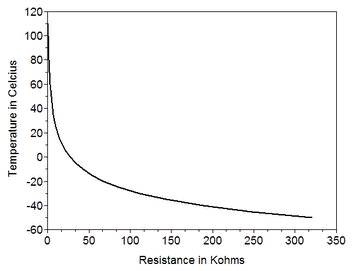The manufacturer of the thermistor provides a specification of this curve. We’ve built that constant into our helper library, so all your code needs to do is compute a logarithm. These calculations are done in Kelvin, so we also provide a helper function to convert Kelvin to Celsius. Add these two functions to your program  (still above the setup function).

Once you have these functions added, modify the loop to print the results of each conversion using the code below.

Upload this to your Arduino and open the serial monitor to see the output (similar to that below). Use ice to cool the thermistor or hot water to heat it (being careful not to get the Arduino wet).

## Part 3: Rainbows

Now that we can successfully read the temperature, we need to translate this to the most appropriate rainbow color. There are many ways to quantify color, and for this purpose the hue-saturation-value (HSV) model is convenient because the colors appear in rainbow order as we vary the hue. In this model the color space is a cylinder (shown below) where the hue is interpreted as the angle around the cylinder.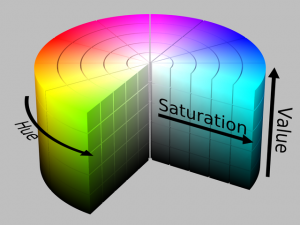The following plot shows the RGB color components as the hue changes.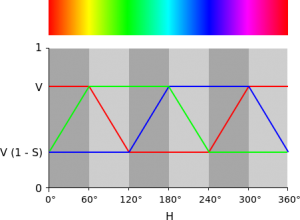So, we need to convert our temperature into an angle in the HSV color space to determine the color. We will map the temperatures 50 C – 0 C onto the angles from 0 (red) to 240 (blue). These calculations are done using integers, so to make it smoother we use 10th’s of a degree. Add the following code to the end of your loop function.

You can upload and run and you will see the computed hue angle for 24 C is about 124 degrees which is right in the middle of the green range.

Finally, we can light up the LED, start by adding the RGB LED to your circuit using pins D3 – D6. On one pin the lead is longer, this is the common pin. Ensure the common pin goes into D4. This will orient your LED correctly. To limit the brightness will will add the 100 Ohm resistor (brown-black-brown-gold bands) from D4 to D7. This arrangement is shown below.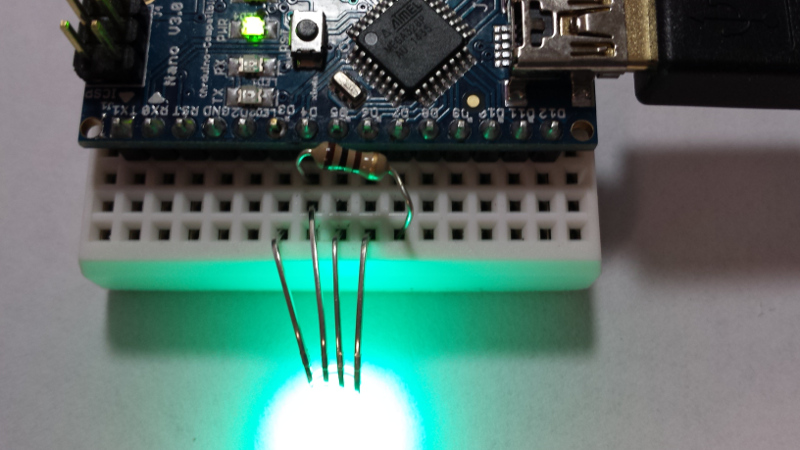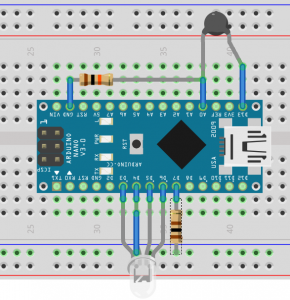To light up the LED we need to compute the RGB (red-green-blue) components of our HSV color. This is beyond the scope of our introduction so, we’ll use a publicly available function to do just this. Copy and paste this code into the end of your source file (after the loop function).

Now add this code to the end of your loop (just after you compute the value for hue) to convert the HSV to RGB and output the values on the RGB LED pins.

Upload your program and you LED will indicate the temperature!

You can follow any responses to this entry through the RSS 2.0 Responses are currently closed, but you can trackback.